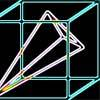#### You may also likeA box of size a cm by b cm by c cm is to be wrapped with a square piece of wrapping paper. Without cutting the paper what is the smallest square this can be?### Plane to See

P is the midpoint of an edge of a cube and Q divides another edge in the ratio 1 to 4. Find the ratio of the volumes of the two pieces of the cube cut by a plane through PQ and a vertex.### Four Points on a Cube

What is the surface area of the tetrahedron with one vertex at O the vertex of a unit cube and the other vertices at the centres of the faces of the cube not containing O?

# Cheese Cutting

##### Age 16 to 18 Challenge Level:

We had lots of solutions suggesting that it is possible to make 16 pieces of cheese. This isn't quite right because you cannot cut all of your 8 pieces in half with one straight cut, although it is a good start because we know that we cannot get more pieces than this. How many do you think will have to be left out from the cut?

Duncan from QEGS Horncastlemade a brave attempt at the problem using extensions of Euler's formula for the edges and vertices of a polyhedra, but, unfortunately, this didn't quite work out correctly -- however, we were still very impressed by this analysis which indicates great mathematical promise and using topology was a clever idea .

Jason correctly realised that you couldn't cut through each piece each time and suggested that 12 was the maximum.However, we think that more pieces are possible.

Well, we had to wait a while, but two excellent solutions were finally given to this tricky problem. Very well done!

ChanWC from Hong Kong suggested reducing the problem to lower dimension as follows
To reduce the 3D case into lower dimension so that we can see the pattern.
for 0-Dimension, no matter how it is cut, the point can not be divided.
for 1-Dimension, the line can only be cut one segment each time, so each cut increases the number of pieces by 1.
for 2-Dimensions, a plane is cut by line. For each non-parallel line cutting the plane, it can cut the previous lines at most one time summary:
cut time 1 2 3 4 5 6 7 8 9 ... n
• 0-DIM 1 1 1 1 1 1 1 1 1 ... 1
• 1-DIM 1 2 3 4 5 6 7 8 9 ... n
• 2-DIM 1 2 4 7 11 16 22 29 37 ... n(n-1)/2 + 1

from the above pattern, i found that the (x-1) DIM of n cut + x DIM of n cut = x DIM of n+1 cut by using this observation, i get this
• 3-DIM 1 2 4 8 15 26 42 64 93 ...

Pippa and Mike from South Hunsley provided this solution
Firstly we looked at the 2-D version of this problem.
We discovered that the greatest number of regions that could be created happened when each existing line was cut by the new line. This new line could not cut at the intersection of existing lines.
This means that the number of regions increases by the number of existing lines +1. This is because if a new line cuts through n lines it cuts through n+1 regions. This will create n+1 new regions.
The sequence we generated is shown below
• No. Cuts 0 1 2 3 4 5 6
• No. Regions 1 2 4 7 11 16 22

• 1/2x^2 + 1/2x + 1

Moving on to the 3-D case, we assumed that the greatest number of pieces were formed by cutting through all the existing planes without cutting through ti intersection of three existing planes.
We then thought that the cross section formed by this latest cut would be the same as the 2-d model with the same number of cuts. So if we were putting in the 4th cut it would pass through 7 pieces of cheese, (the number of regions for 3 cuts on the 2-D model) so our new sequence is generated by increasing each term by the terms in the 2-D sequence.
This gives
• No. Cuts 0 1 2 3 4 5 6
• No. Pieces 1 2 4 8 15 26 42

This gives the cubic formula
• 1/6x^3 + 5/6x + 1

So 10 cuts gives 176
50 cuts gives 20876
100 cuts gives 166751